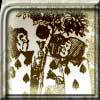#### You may also like### Card Trick 2

Can you explain how this card trick works?### Painting Cubes

Imagine you have six different colours of paint. You paint a cube using a different colour for each of the six faces. How many different cubes can be painted using the same set of six colours?### Cube Paths

Given a 2 by 2 by 2 skeletal cube with one route `down' the cube. How many routes are there from A to B?

# Cunning Card Trick

##### Age 11 to 14Challenge Level

Well done Sara and Terence for cracking this Cunning Card Trick.

Sara started:

"My solution starts from when you have the three piles plus the magic pile.

Of the three piles, take the three bottom cards to be $x$, $y$ and $z$.
In the $x$ pile there will be $13 - x + 1$ cards.
This is because whatever the value of $x$, you counted on to $13$, (if $x = 7$ you count 8, 9, 10, 11, 12, 13 (6 cards)) but you also have the $x$ card which is why you add 1 more.
So an $x$ value of $7$ gives you $7$ cards in the pile, $8$ gives you $6$, queen gives you $2$, etc.

So pile $x$ contains $14 - x$ cards, pile $y$ contains $14 - y$ cards and pile $z$ gives you $14 - z$ cards."

Using the fact that there are 52 cards in a pack, Terence finished off the argument as follows:

"So, we have in total $42 - (x + y + z)$ cards in the piles, and so $10 + x + y + z$ cards in the magic counting pile.

Suppose we deal y and z cards out, there will be just $10 + x$ cards left in the magic counting pile.
Dealing a further 10 leaves $x$ cards, which is the value of the bottom card of the secret pile!

Secret revealed!!!"

Alexander and James from The Batt Primary School, Witney have just sent us this solution
Many thanks for such a clear explanation.